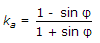# Civil Engineering - Soil Mechanics and Foundation Engineering

### Exercise :: Soil Mechanics and Foundation Engineering - Section 4

41.

If e0, e, σ', σ0' have their usual meanings, the coefficient of compressibility (ac), is given by

 A.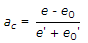B.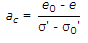C.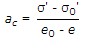D.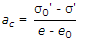Explanation:

No answer description available for this question. Let us discuss.

42.

A saturated soil sample has water content of 40% and specific gravity of soil particles 2.7. The void ratio of the soil, is

 A. 0.4 B. 0.52 C. 1.08 D. none of these.

Explanation:

No answer description available for this question. Let us discuss.

43.

For testing a saturated clay for shear strength, the test recommended, is

 A. direct shear test B. triaxial compression test C. unconfined compression test D. all the above.

Explanation:

No answer description available for this question. Let us discuss.

44.

The critical exist gradient of seepage water in soils, increases with

 A. an increase in specific gravity B. a decrease in specific gravity C. a decrease in void ratio D. both (a) and (c) E. none of these.

Explanation:

No answer description available for this question. Let us discuss.

45.

The coefficient ka of the active earth pressure, is given by

 A.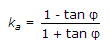B.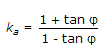C.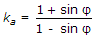D.